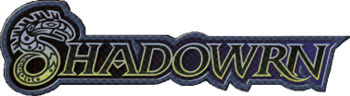Back to the main page

# Mailing List Logs forFrom: Rat winterhawk@*********.net Announcing the 3rd Annual Magespace Short Story Contest Wed, 16 Aug 2000 14:26:12 -0700

The 3rd Annual Magespace Short Story Contest is now underway.
This year there are *two* contests, along with a whole
heap o' prizes waiting to be won! This year's prizes include:

- A copy of "Wolf and Raven" signed by Mike Stackpole
- Original art by Fred Hooper (2 of them)
- 5 of the 7 2057 Election badges
- A copy of "Matrix" signed by Mike Mulvihill, Fred Hooper,
and Steve Kenson

Bop on by the Magespace for full details. The URL's in
the .sig. Deadline for entries is October 31.

Thanks, and good luck to all who enter!
--Rat

=>=>=>=>=>=>=>=>=>=>=>=>=>=>=>=>=>=>=>=>=>=>=>=>=>=>=>=>=>=>=>=>
Rat - winterhawk@*********.net http://www.magespace.net
Winterhawk's Virtual Magespace - Shadowrun Fiction and More!
DOD#1211 1999 K1200RS - "Dunkelzahn"
"The pickles are staring at me..."
<=<=<=<=<=<=<=<=<=<=<=<=<=<=<=<=<=<=<=<=<=<=<=<=<=<=<=<=<=<=<=<